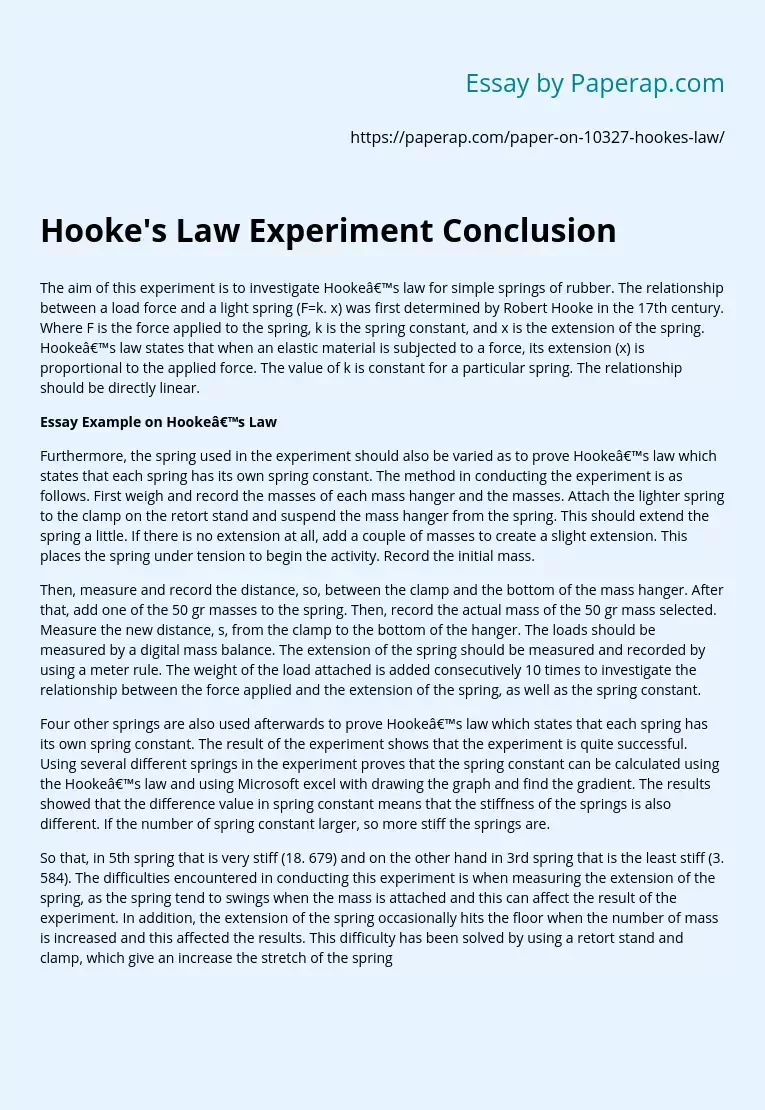# Hooke's Law Experiment Conclusion

The aim of this experiment is to investigate Hooke’s law for simple springs of rubber. The relationship between a load force and a light spring (F=k. x) was first determined by Robert Hooke in the 17th century. Where F is the force applied to the spring, k is the spring constant, and x is the extension of the spring. Hooke’s law states that when an elastic material is subjected to a force, its extension (x) is proportional to the applied force.

The value of k is constant for a particular spring. The relationship should be directly linear.

## Essay Example on Hooke’s Law

Furthermore, the spring used in the experiment should also be varied as to prove Hooke’s law which states that each spring has its own spring constant. The method in conducting the experiment is as follows. First weigh and record the masses of each mass hanger and the masses. Attach the lighter spring to the clamp on the retort stand and suspend the mass hanger from the spring.

This should extend the spring a little. If there is no extension at all, add a couple of masses to create a slight extension. This places the spring under tension to begin the activity. Record the initial mass.

Then, measure and record the distance, so, between the clamp and the bottom of the mass hanger. After that, add one of the 50 gr masses to the spring. Then, record the actual mass of the 50 gr mass selected. Measure the new distance, s, from the clamp to the bottom of the hanger.

Get quality help nowDoctor JenniferVerified

Proficient in: Experiment5 (893)

“ Thank you so much for accepting my assignment the night before it was due. I look forward to working with you moving forward ”+84 relevant experts are online

The loads should be measured by a digital mass balance. The extension of the spring should be measured and recorded by using a meter rule. The weight of the load attached is added consecutively 10 times to investigate the relationship between the force applied and the extension of the spring, as well as the spring constant.

Four other springs are also used afterwards to prove Hooke’s law which states that each spring has its own spring constant. The result of the experiment shows that the experiment is quite successful. Using several different springs in the experiment proves that the spring constant can be calculated using the Hooke’s law and using Microsoft excel with drawing the graph and find the gradient. The results showed that the difference value in spring constant means that the stiffness of the springs is also different. If the number of spring constant larger, so more stiff the springs are.

So that, in 5th spring that is very stiff (18. 679) and on the other hand in 3rd spring that is the least stiff (3. 584). The difficulties encountered in conducting this experiment is when measuring the extension of the spring, as the spring tend to swings when the mass is attached and this can affect the result of the experiment. In addition, the extension of the spring occasionally hits the floor when the number of mass is increased and this affected the results. This difficulty has been solved by using a retort stand and clamp, which give an increase the stretch of the spring but still easily adjusted.

In conclusion, it could be said that the experiment is successful in verifying value of the spring constant. Both the Hooke’s law and the graph give similar result, thus proving the hypothesis. My suggestion to improve the experiment is to carefully measure the extension of the spring despite the variation of the spring. This is best dealt with by carefully observed the spring until it places perfectly so that there will be no further movements that may lead to the mistake in calculating the exact extension of the spring.